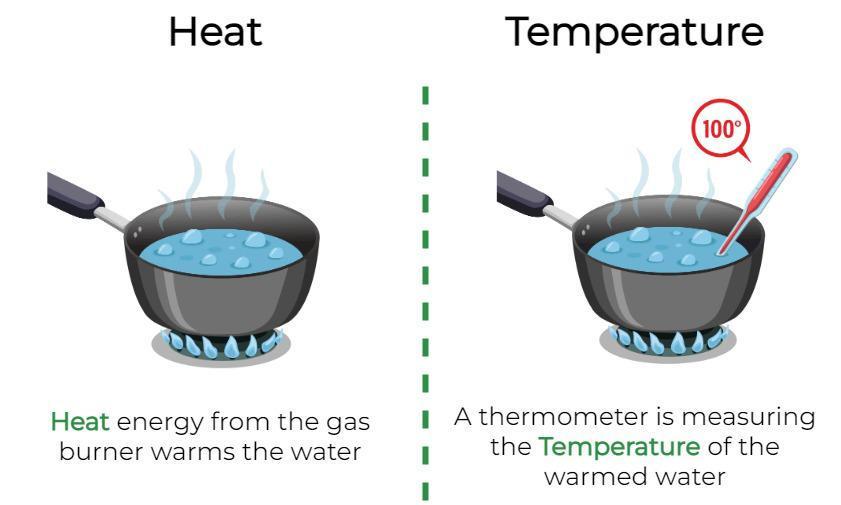GFG App
Open AppBrowser
Continue

# Difference between Heat and Temperature

Heat and Temperature are two related terms that people may confuse often. It should be noted that Heat and temperature are two different quantities. The fundamental difference between heat and temperature is that Heat is the form of energy that transfers from a hot state to a cold state. The unit of Heat is Joule. However, the temperature is the measure of the hotness and coldness of an object. The SI unit of Temperature is Kelvin.

As shown below, water is boiling on a gas burner in a utensil. It can be observed that heat energy is transferred from the gas burner to the water when boiled. The water gets boiled at 100 °C, which is its temperature, and is measured with the help of a thermometer.## Heat

Heat is a form of energy that is transferred between two bodies because of a temperature difference existing between them.

Heat is the term used to describe the thermal energy that is transferred between molecules in a system due to a temperature difference. Joules are used to measure it. An object cannot have heat; it can only gain or lose heat. Heat is a measure of change rather than a property that an object or system possesses.

Atoms and molecules make up everything in the universe. Kinetic energy is created when molecules and atoms in a body move, and heat is generated when they meet.

For example, when we fill a container with water and place it on the stove, the container heats up. The reason for this is that water molecules flow in all directions equally and clash with one another, causing the water’s temperature to rise and grow hotter.

### SI Unit of Heat

SI unit of Heat is Joule (J) and can also be measured in Calories (Cal). Both units are related as,

1 Cal = 4.186 J

### Heat Transfer

Heat can transfer between different bodies or between different body parts. The study of methods and processes for transferring thermal energy is referred to as “heat transfer.” For heat to travel between two bodies, there must be a temperature differential. These bodies must be at two different temperatures, one higher than the other, in order for heat to transfer from one to the other.

Processes of Heat Transfer are:

1. Conduction
2. Convection

## Temperature

Temperature is a measure of the degree of hotness or the intensity of sensible heat. Whenever two bodies at different temperatures are brought into thermal contact, heat flows from one at a higher temperature to the other at a lower temperature.

Temperature may be defined as the thermal condition of a body that determines the direction of heat flow i.e. it determines whether the body will receive heat from another body or it will give heat to the same.

The human body is quite sensitive to changes in temperature. But the sense of touch is purely qualitative. while scientific experiment requires that every physical quantity should be measurable. Instruments which is used to measurement of temperature are called thermometers.

### SI Unit of Temperature

The SI unit of temperature is Kelvin (K). Other units of temperature are °C and °F and the three temperature scales are the Fahrenheit scale, Celsius scale, and Kelvin scale.

### Measurements of Temperature

Because molecules are such tiny particles, we must use an indirect method to estimate their kinetic energy. When a substance is heated, the molecules within it move more quickly. The increased mobility caused most material volume or space to slightly increase (motion). The expansion of a material is used by thermometers as a proxy for temperature measurement.

## FAQs on Heat and Temperature

Question 1: What is Heat?

Heat is the term used to describe the thermal energy that is transferred between molecules in a system due to a temperature difference. Joules are used to measure it.

Question 2: What is Temperature?

Temperature may be defined as the thermal condition of a body that determines the direction of heat flow i.e. it determines whether the body will receive heat from another body or it will give heat to the same.

Question 3: What are the Units of Measurement of Heat and temperature?

The unit of Heat is Joule (J) and can also measured in Calories (Cal). And the unit of temperature is Kelvin (K). Other units of temperature are °C and °F.

Question 4: What is the main difference between heat and temperature?

Heat is a form of energy which can be transferred from a hot to a cool temperature object. However, the temperature of an object measure the degree of hotness or coolness.

Question 5: How to convert Fahrenheit to Celsius?

Fahrenheit to Celsius can be using the following relation:

TC = (TF – 32)

where

• TC is the Temperature in Fahrenheit and
• TF is the Temperature in Celsius.

### Related Articles

My Personal Notes arrow_drop_up